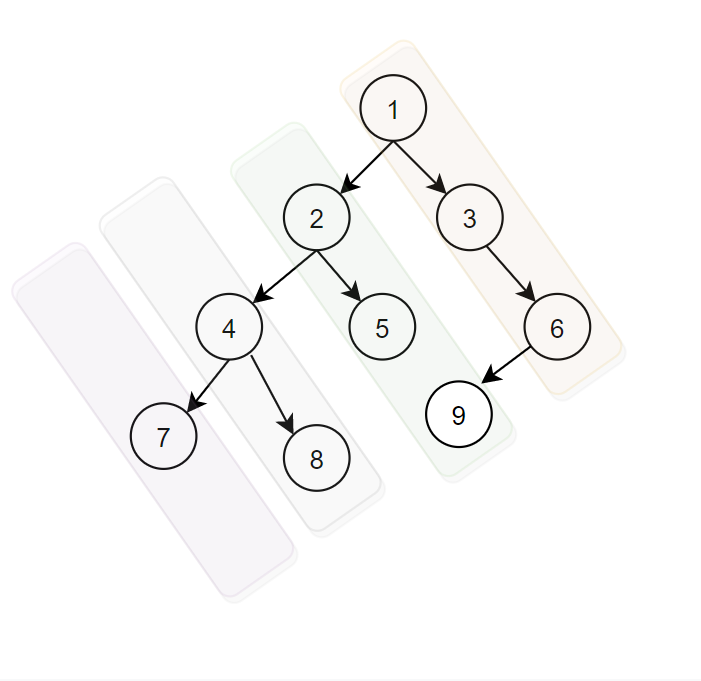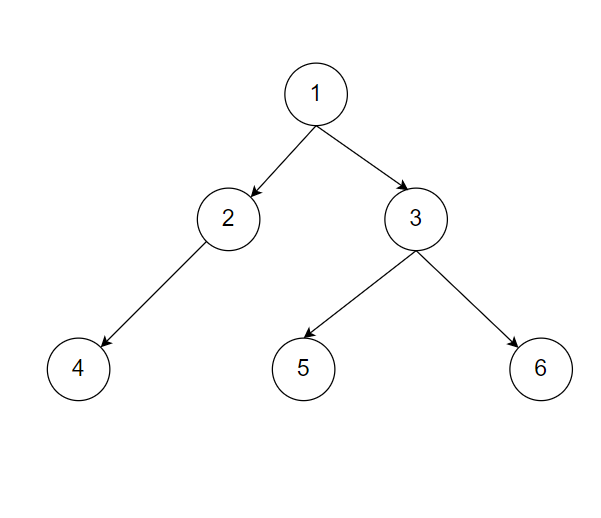Diagonal Traversal

Posted: 20 Nov, 2020
Difficulty: Moderate

PROBLEM STATEMENT

Note:

Order of return of diagonal path’s array/vector: The rightmost diagonal path must come first, and so on.
Every parent node comes first then the child node. In other words, return the diagonal element from top to bottom.

Example

Consider the given binary tree.There are 4 diagonal paths:
1 3 6
2 5 9
4 8
7
You need to return ‘1 3 6 2 5 9 4 8 7’.

Let's consider this exampleDiagonal paths are:
1 3 6
2 5
4

You need to return ‘1 3 6 2 5 4’.
Input Format:
The first line of input contains an integer ‘T’ denoting the number of test cases.
The next ‘T’ lines represent the ‘T’ test cases.

The first line of input contains the elements of the tree in the level order form separated by a single space.
If any node does not have a left or right child, take -1 in its place. Refer to the example below.

Example:

Elements are in the level order form. The input consists of values of nodes separated by a single space in a single line. In case a node is null, we take -1 in its place.

For example, the input for the tree depicted in the below image would be :1
2 3
4 -1 5 6
-1 7 -1 -1 -1 -1
-1 -1

Explanation :
Level 1 :
The root node of the tree is 1

Level 2 :
Left child of 1 = 2
Right child of 1 = 3

Level 3 :
Left child of 2 = 4
Right child of 2 = null (-1)
Left child of 3 = 5
Right child of 3 = 6

Level 4 :
Left child of 4 = null (-1)
Right child of 4 = 7
Left child of 5 = null (-1)
Right child of 5 = null (-1)
Left child of 6 = null (-1)
Right child of 6 = null (-1)

Level 5 :
Left child of 7 = null (-1)
Right child of 7 = null (-1)

The first not-null node (of the previous level) is treated as the parent of the first two nodes of the current level. The second not-null node (of the previous level) is treated as the parent node for the next two nodes of the current level and so on.

The input ends when all nodes at the last level are null (-1).
Note :
The above format was just to provide clarity on how the input is formed for a given tree.

The sequence will be put together in a single line separated by a single space. Hence, for the above-depicted tree, the input will be given as:

1 2 3 4 -1 5 6 -1 7 -1 -1 -1 -1 -1 -1
Output Format:
For every test case, the output prints in a single line the diagonal paths of the given binary tree.

The output of each test case will be printed in a separate line.
Note:
You do not need to print anything; it has already been taken care of. Just return an array/list of all diagonal paths in which the rightmost diagonal path comes first, the second rightmost diagonal path comes second, and so on.
Constraint :
1 <= T <= 10^2
1 <= N <= 3*10^3
-10^9 <= DATA <= 10^9

Where ‘N’ is the number of nodes in the tree, and 'DATA' denotes data contained in the node of a binary tree.

Time Limit: 1 secApproach 1
1. The idea is to use a Hash map called ‘LEVELMAP’.
2. We use different levels and use them as keys in the map.
3. The value of each key in the map is the vector/list of nodes.
4. In the end, We return values from the map.
5. Suppose the level of current node ‘CURNODE’ is ‘l’
6. Then, the level of the right child will be ‘l’, and the level of the left child will be ‘l+1’, and ‘LEVELMAP[l] =CURNODE.DATA’
7. And proceed the same steps for ‘LEFT’ child with level  ‘l+1’ and ‘RIGHT’ child with the level of ‘l.’
8. Let’s consider this binary tree.

1. Observe the level of binary tree
• Level 0 => [1,3,6]
• Level 1 => [2,5]
• Level 2 => 
• LEVELMAP = {}
• Consider starting level for ‘1’ is 0
• Store level in such a way that
• LEVELMAP = { 0:[1,3,6], 1:[2,5], 2: }
2. At the end, We return value in such a form => 1 3 6 2 5 4.